# Multiplication by3 Digit Numbers

## NUMBERS AND OPERATIONSThe multiplication is that operation that consists of calculating the product (result) that is obtained by adding the same number (factor or multiplicand) so many times as the value of the other number (factor or multiplicator or multiplier).

Multiplication is used when we have to replace the calculation of certain repetitive additions (for example: 2 + 2 + 2 + 2 = 8) by a more efficient method (2 x 4 = 8).

In general, multiplication is considered the inverse operation of division.

MULTIPLY TABLES FROM 1 TO 10

We have prepared a template with the multiplication tables from 1 to 10 ready to download and print so that you can consult them when you need it and help you learn them quickly.

PYTHAGORAS MULTIPLY TABLES

We have also prepared a complete Pythagorean table where the multiplication tables from 1 to 10 are represented to facilitating very visual learning using patterns and symmetries and stimulate logical reasoning among students.

#### Know the terms of multiplication

Do you know what multiplications are and what are they for? Can you tell what its symbol "x" means? Do you know the differences between the multiplicand, the multiplier and the product ? Don't worry, through the lesson we explain each of the elements of multiplication.

SPANISHENGLISHThe elements of multiplication (multiplicand and multiplier) that are multiplied together are called "factors" or "coefficients":

- The multiplicand is the element that tells us "how many times" we are going to add or multiply the multiplier digit (in this example, it is 24). It is at the top of any multiplication.

- The multiplier represents the element that multiplies each of the numbers in the multiplicand and we will take as a reference its multiplication table (in this case, the table of 7). It is located at the bottom of the multiplication and is always written to the right of the multiplication sign ("x")

- The multiplication sign determines the multiplicative operation and is read "multiplied by" or "times", in the following example, it would be "24 multiplied by 7" or "24 times 7". It is represented by a cross "x" (relative to the Cross of Saint Andrew) or by using a point at half height (•)

- The equal sign is the equality symbol and is read "equals". In our example, we will read the multiplication as "24 times 7 equals 168"

- The product contains the result of applying the multiplication algorithm (24 x 7 = 168)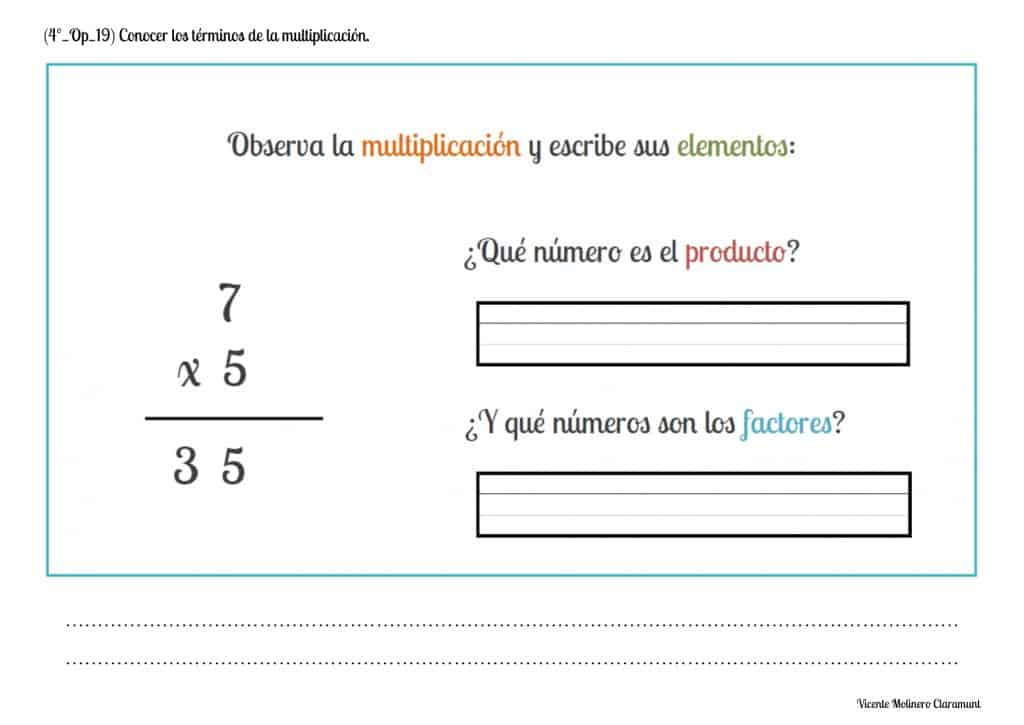#### Recognize the concept of multiplication as a repeated addition of equal addends

We must understand that, mathematically, multiplying a certain quantity by a number consists of adding said quantity as many times as the number indicates.

In other words, multiplications are those mathematical operations that are carried out to calculate the result (also called "product") of adding the same number ("multiplicand") so many times as indicated by the other number ("multiplier").

We present you some printable PDF activities accompanied by their explanation and an educational game so that you understand that all multiplication is equivalent to the repeated addition of equal addends.

SPANISHENGLISHIn the following example we can see that multiplying 5 times 3 (that is 5x3) is also equivalent to adding 3 times the number 5 (meaning 5+5+5), obtaining in both cases the same result (product):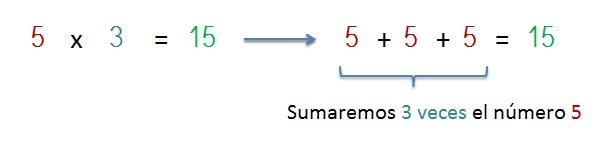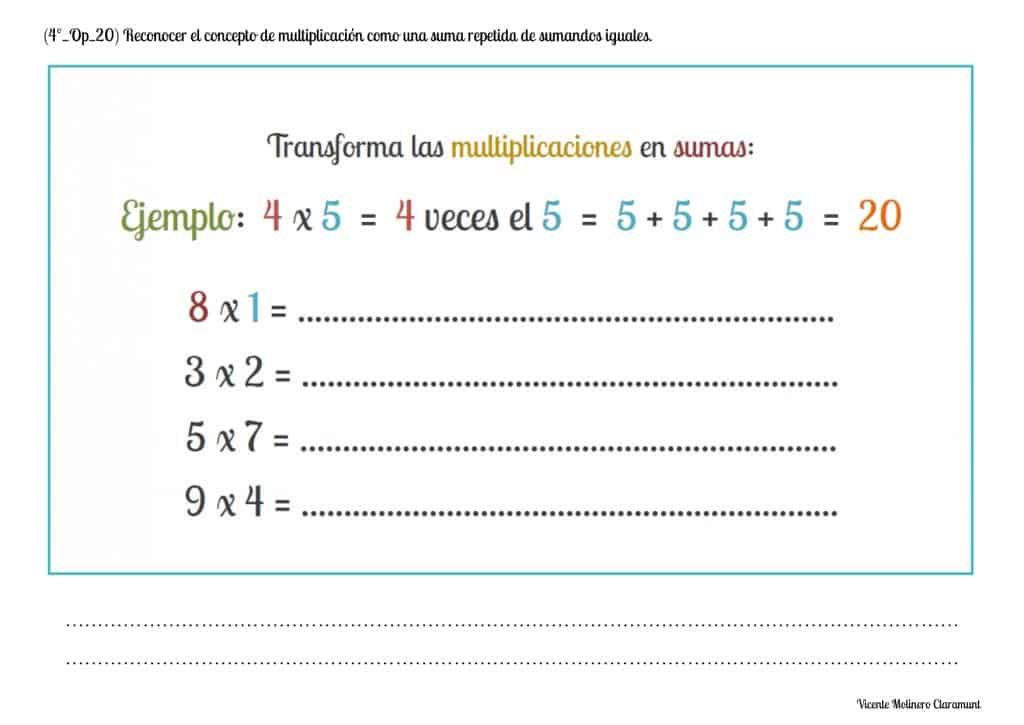#### Multiply natural numbers without carrying: 4 digits by 3 digits

We present you some educational worksheets in PDF format accompanied by their explanation and an online game so that you can learn to solve the four-digit multiplications (multiplicand) by three digits (multiplier).

SPANISHENGLISHLook at the solved multiplication that we propose and you will learn how to calculate a multiplication of four digits in the multiplicand by three digits in the multiplier without regrouping.

LET'S MULTIPLY 5,132 x 1231st) We start by multiplying the ones of the multiplier (table of 3) from right to left and for each of the multiplicand digits:

3 x 2 = 6 ones

3 x 3 = 9 tens

3 x 1 = 3 hundreds

3 x 5 = 15 thousands

We will write the results below the horizontal dividing line and aligning them according to their place value from the ones.

2nd) We start a new line and write a zero to the right to align the figures from the tens, we will repeat the process of multiplying again from right to left.

3rd) We will multiply the tens of the multiplier (table of 2) by each of the digits of the multiplicand:

2 x 2 = 4 ones

2 x 3 = 6 tens

2 x 1 = 2 hundreds

2 x 5 = 10 thousands

We will write the results below the horizontal dividing line and aligning them according to their place value from the tens.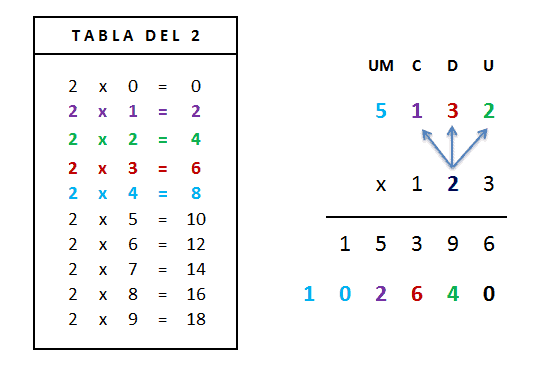4th) We start a new line and write two zeros to the right to align the figures from the hundreds, we will repeat the process of multiplying again from right to left.5th) We continue multiplying the hundreds of the multiplier (table of 1) from right to left and for each of the multiplicand digits:

1 x 2 = 2 ones

1 x 3 = 3 tens

1 x 1 = 1 hundred

1 x 5 = 5 thousands

Again we will write the results below the horizontal line and align them according to their place value from the hundreds.

6th) We will write a horizontal dividing line, we will draw the sign of the addition (+) and then we will add the products obtained with the previous multiplications from right to left.

Finally, we will write the result of the sum below the horizontal dividing line.

You've got it! The solution: 5,132 x 123 = 631,236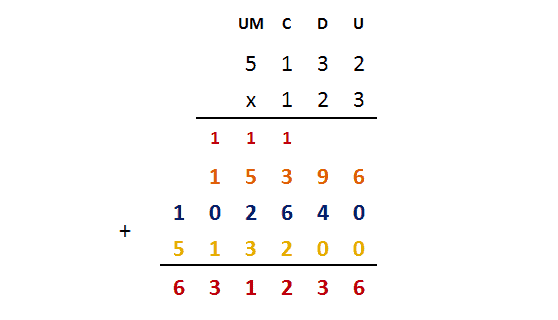#### Solve multiplication word problems without carrying with natural numbers: 4 digits x 3 digits

We offer you a series of multiplication word problems of four figures by three figures (without regrouping) for you to continue improving. We also provide you with video lessons where we explain in a simple way what is the procedure for its resolution. Time to practice!

SPANISHENGLISH#### Multiply natural numbers with carrying: 4 digits by 3 digits

We present you some downloadable and printable exercises accompanied by their pedagogical lesson and an interactive activity so that you can learn to easily solve the multiplications by carrying of 4-digits (multiplicand) by 3-digits (multiplier).

SPANISHENGLISHWe show you a solved example that will help you learn step by step how to solve 4-digits multiplication in the multiplicand by 3-digits in the multiplier and regrouping.

LET'S MULTIPLY 3,869 x 475

1st) We start by multiplying the ones of the multiplier (table of 5) from right to left and for each of the multiplicand digits:

If the result of the multiplication of two digits is equal to or greater than 10 (for example, 8 x 4 = 32), we will move the digit on the left (in this example it would be 3) to the next column to add it to the result of the next multiplied number.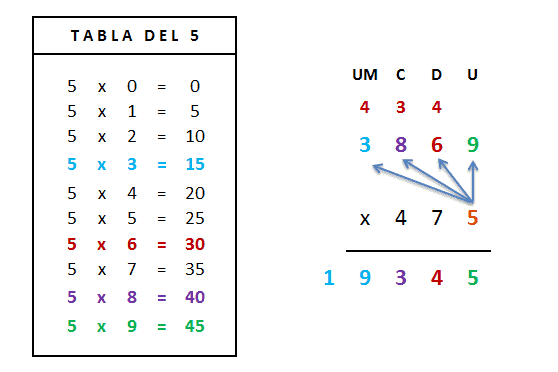5 x 9 = 45 ones, that is, 4 tens and 5 ones

5 x 6 = 30 tens + 4 tens = 34 tens, that is, 3 hundreds and 4 tens

5 x 8 = 40 hundreds + 3 hundreds = 43 hundreds, that is, 4 thousands and 3 hundreds

5 x 3 = 15 thousands + 4 thousands = 19 thousands

2nd) We start a new line and write a zero to the right to align the figures from the tens, we will repeat the process of multiplying again from right to left.

3rd) We will multiply the tens of the multiplier (table of 7) by each of the digits of the multiplicand:

Remember, if the result of each multiplication is greater than or equal to 10, we will move the digit on the left to the next column to add it to the result of the next multiplication.

7 x 9 = 63 ones, that is, 6 tens and 3 ones

7 x 6 = 42 tens + 6 tens = 48 tens, that is, 4 hundreds and 8 tens

7 x 8 = 56 hundreds + 4 hundreds = 60 hundreds, that is, 6 thousands and 0 hundreds

7 x 3 = 21 thousands + 6 thousands = 27 thousands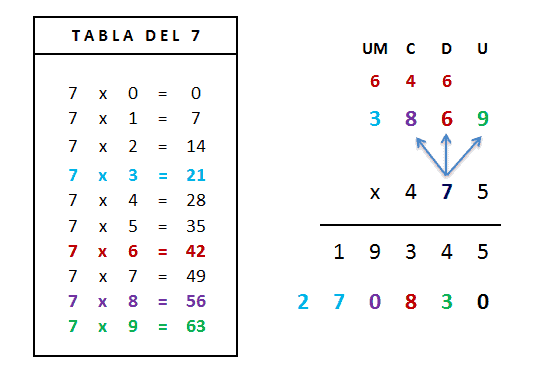4th) We start a new line and write two zeros to the right to align the figures from the hundreds, we will repeat the process of multiplying again from right to left.

5th) We will multiply the hundreds of the multiplier (table of 4) by each of the figures of the multiplicand.

And don't forget it! If the result of each multiplication is greater than or equal to 10, we will move the digit on the left to the next column to add it to the result of the next multiplication.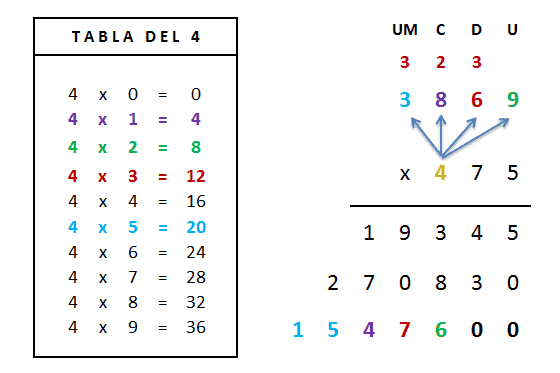4 x 9 = 36 ones, that is, 3 tens and 6 ones

4 x 6 = 24 tens + 3 tens = 27 tens, that is, 2 hundreds and 7 tens

4 x 8 = 32 hundreds + 2 hundreds = 34 hundreds, that is, 3 thousands and 4 hundreds

4 x 3 = 12 thousands + 3 thousands = 15 thousands

6th) We will write a horizontal dividing line, we will draw the sign of the addition (+) and then we will add the products obtained with the previous multiplications from right to left.

Finally, we will write the result of the sum below the horizontal dividing line.

You've got it! The solution: 3,869 x 475 = 1,837,775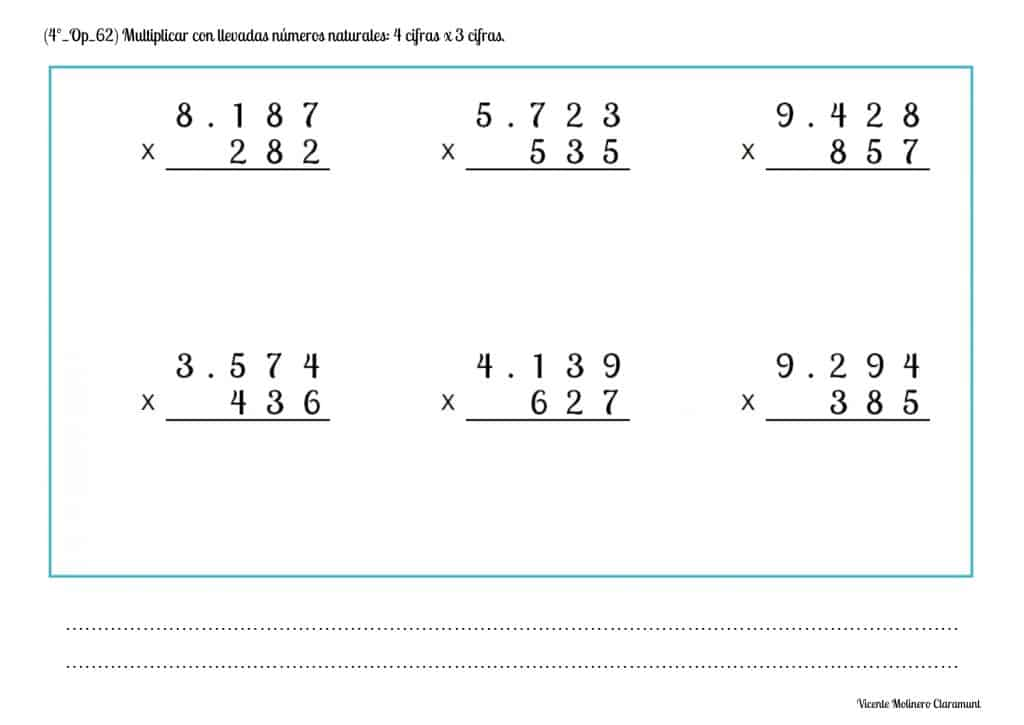#### Solve multiplication word problems with carrying with natural numbers: 4 digits x 3 digits

We propose you our multiplication word problems of four figures by three figures (regrouping) so that you continue to improve. In addition, we provide you with video lessons where we explain in a practical way what is the procedure for its correct resolution.

SPANISHENGLISH#### Multiply natural numbers by 10, 100, 1,000 and so on

Do you know how to multiply a number by the unit followed by zeros? We will explain it to you below!

First, watch the video explanation and learn with simple steps to multiply any natural number by the unit followed by zeros: 10, 100, 1,000, 10,000, 100,000 and so on.

SPANISHENGLISHThe procedure is very simple: only as many zeros are added to the end as there are in the number we have multiplied by, as shown in the examples.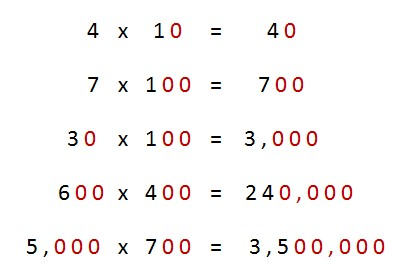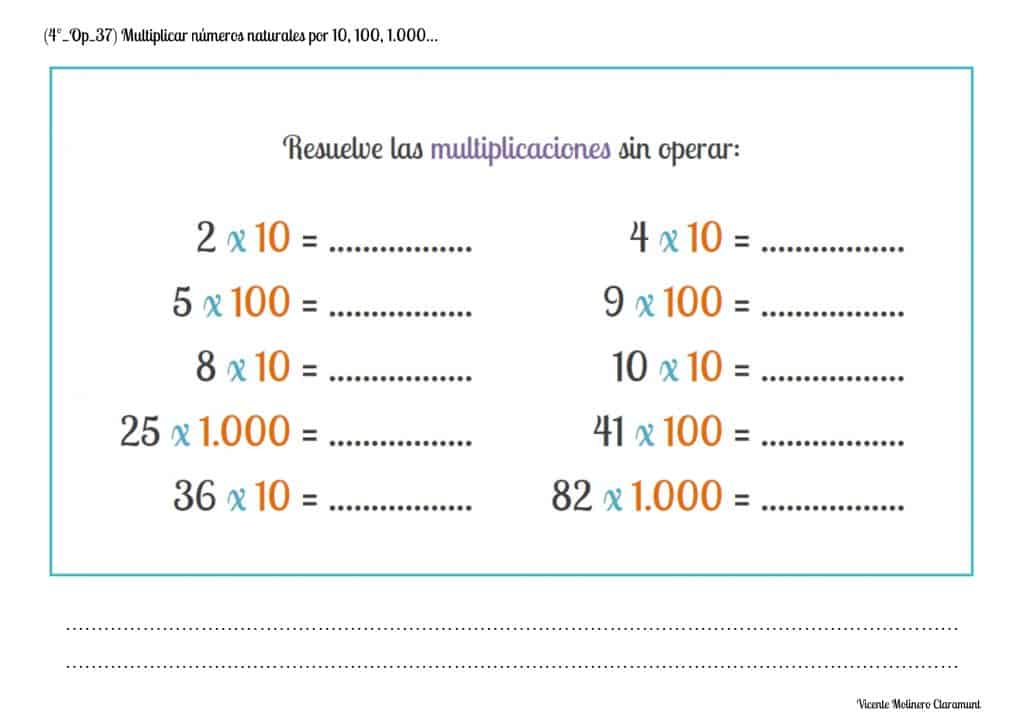#### Mentally calculate simple operations: Multiplication

Here are some mental math activities to test your memory and show that you already know all the multiplication tables.#### Applying the Commutative Property of Multiplication

One of the most important fundamental properties of multiplication is "commutativity":

A x B = B x A

The Commutative Property of Multiplication states that "the order in which the factors are multiplied does not alter the product".

In other words, when we multiply two numbers (factors) with each other, the order in which they are multiplied will not matter because the result (product) will always be the same.

SPANISHENGLISH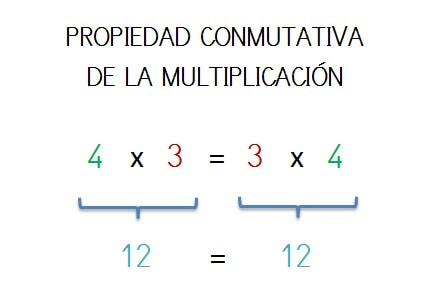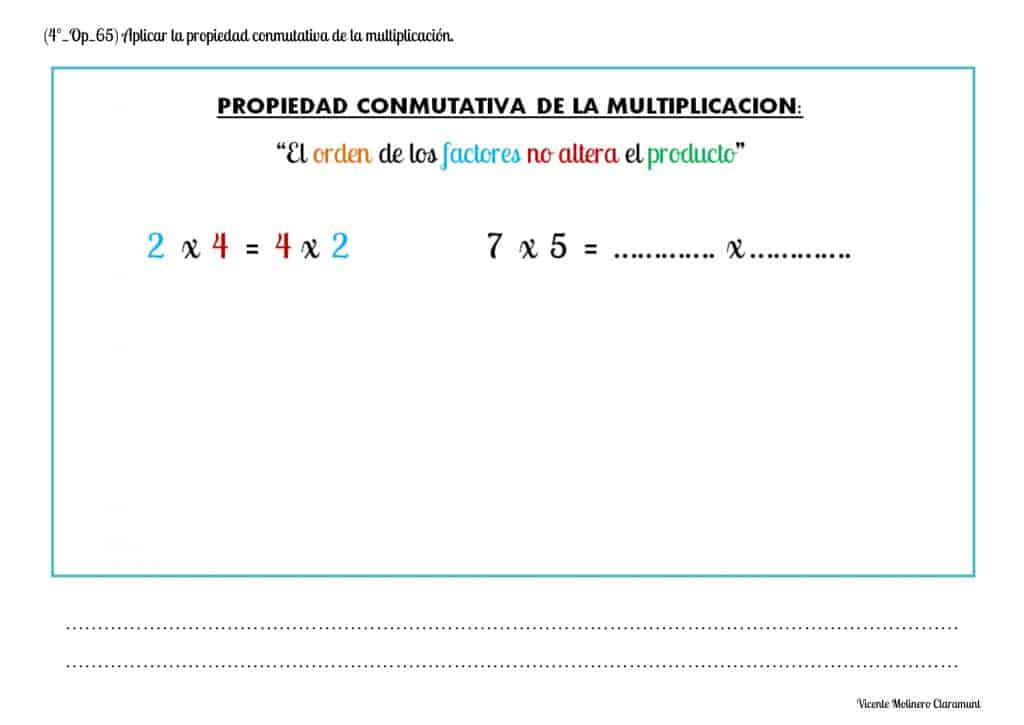#### Apply the Associative Property of Multiplication

Another of the fundamental properties of multiplication is "associativity", where the use of parentheses tells us in what order we must solve the multiplications :

A x ( B x C ) = ( A x B ) x C

The Associative Property of Multiplication determines that "when three or more numbers are multiplied, the result is the same regardless of the order in which the factors are multiplied".

In other words, when we multiply three or more numbers (factors) with each other, the order in which they are multiplied will not matter because the result (product) will always be the same.

SPANISHENGLISH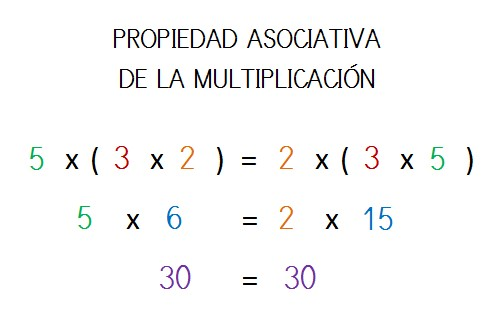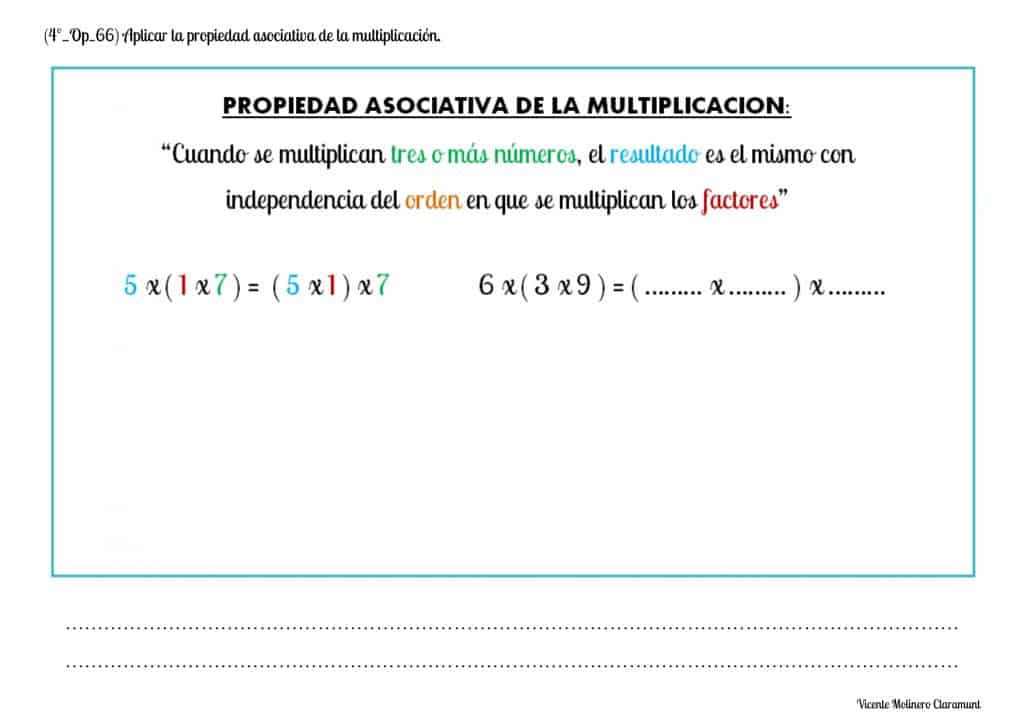Select more exercises, worksheets and activities of Mathematics for each of the 4 learning blocks of the Educational Curriculum ("Numbers and Operations", "Quantities and Measurements", "Geometric and Spatial Reasoning" and "Data Analysis and Probability") aimed at improving the logical-mathematical competencies and skills that are developed throughout "Grade 4" or "Year 5":